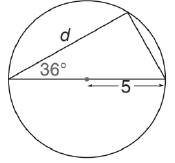Chapter 11.3, Problem 19E### Elementary Geometry for College St...

6th Edition
Daniel C. Alexander + 1 other
ISBN: 9781285195698

#### Solutions

Chapter
Section### Elementary Geometry for College St...

6th Edition
Daniel C. Alexander + 1 other
ISBN: 9781285195698
Textbook Problem
1 views

# In Exercises 15 to 20, use the sine, cosine, or tangent ratio to find the lengths of the indicated sides to the nearest tenth of a unit.To determine

To find:

The lengths of the indicated sides to the nearest tenth of a unit using the sine, cosine or tangent ratio.

Explanation

Given:

The triangle inscribed in the circle with the indicated sides is shown below.

Definition used:

The trigonometric ratios:

Calculation:

Let α=36.

From the figure we see that the radius of the circle is 5.

Therefore the diameter is 25=10.

We know that the angle inscribed in a semicircle is a right angle.

Hence the hypotenuse of the triangle is the diameter of the circle, whose value is 10.

We need to find the value of d.

cos36=d10

Let us use Ti-83 calculator to find the value of cos36.

Press ON in Ti-83 calculator.

Since we need to find the value of degree, we need to change the mode to degrees

### Still sussing out bartleby?

Check out a sample textbook solution.

See a sample solution

#### The Solution to Your Study Problems

Bartleby provides explanations to thousands of textbook problems written by our experts, many with advanced degrees!

Get Started

#### Factor each expression in Problems 7-18 as a product of binomials. 11.

Mathematical Applications for the Management, Life, and Social Sciences

#### Expand each expression in Exercises 122. (y1y)2

Finite Mathematics and Applied Calculus (MindTap Course List)

#### True or False: 23xdx is an improper integral.

Study Guide for Stewart's Single Variable Calculus: Early Transcendentals, 8th

#### Explain the role of voluntary participation in informed consent.

Research Methods for the Behavioral Sciences (MindTap Course List)